# ISEE Lower Level Math : How to identify the parts of a list

## Example Questions

1 2 3 5 Next →

### Example Question #227 : Data Analysis And Probability

Joe's three CDs each have an average of thirteen songs on them. If one CD has twelve songs and another CD has thirteen songs, how many songs does the third CD have on it?Explanation:

The average of a set consists of the sum of the numbers in a set divided by that items in that set.

Therefore, the following equation would apply, in whichis equal to the number of songs on the third CD: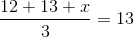Multiply each side by 3.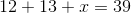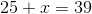Subtract 25 from each side.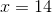### Example Question #228 : Data Analysis And Probability

Which of the numbers in the set below is the smallest?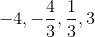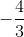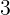Explanation:

The smallest number will be the number that is the most negative. The number -4 is the most negative because it is the furthest away from zero on the number line of negative numbers from the list provided. Therefore, -4 is the correct answer.

### Example Question #229 : Data Analysis And Probability

What are the next three terms in the following set?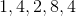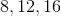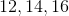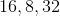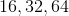Explanation:

You must look at the pattern that is present first.  The first term is multiplied by. Then the next value is divided by. This pattern follows for the third and fourth terms. So it must continue with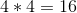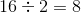, and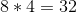.

### Example Question #230 : Data Analysis And Probability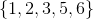What does the above set represent?

All odd numbers belowAll prime numbers belowFactors ofAll outcomes of rolling a die

All outcomes of drawing cards from a standard deck

All outcomes of rolling a die

Explanation:

A die has six sides numbered one through six so the set above must represent that situation.

All prime numbers below: Is false because six is not a prime number.

Factors of: Is false because five is not a factor of.

All odd numbers below: Is false because three of the numbers in the set are even numbers.

All outcomes of drawing cards from a standard deck: Is false because a deck of cards also has face cards and numbers larger than six.

1 2 3 5 Next →